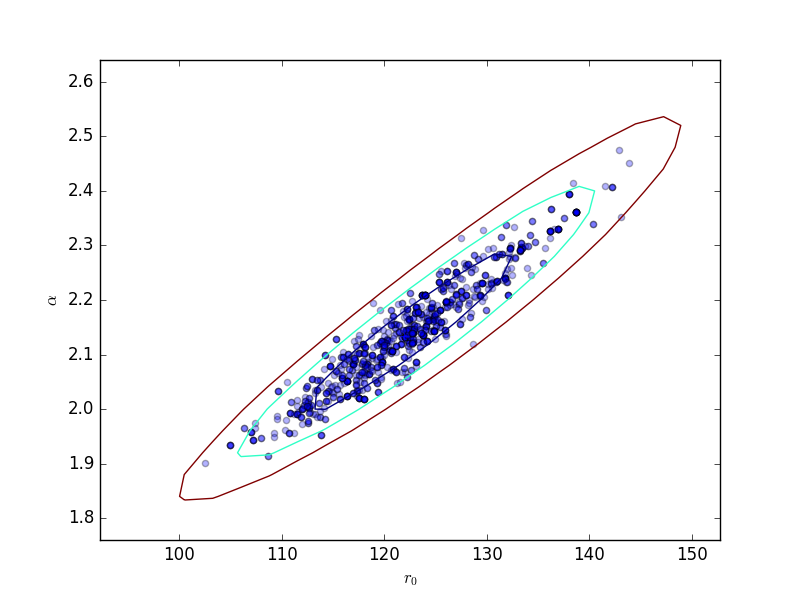# Markov Chain Monte Carlo and Poisson data¶

Sherpa provides a Markov Chain Monte Carlo (MCMC) method designed for Poisson-distributed data. It was originally developed as the Bayesian Low-Count X-ray Spectral (BLoCXS) package, but has since been incorporated into Sherpa. It is developed from the work presented in Analysis of Energy Spectra with Low Photon Counts via Bayesian Posterior Simulation by van Dyk et al.

Unlike many MCMC implementations, idea is that have some idea of the search surface at the optimum - i.e. the covariance matrix - and then use that to explore this region.

## Example¶

Note

This example probably needs to be simplified to reduce the run time

### Simulate the data¶

Create a simulated data set:

>>> np.random.seed(2)
>>> x0low, x0high = 3000, 4000
>>> x1low, x1high = 4000, 4800
>>> dx = 15
>>> x1, x0 = np.mgrid[x1low:x1high:dx, x0low:x0high:dx]


Convert to 1D arrays:

>>> shape = x0.shape
>>> x0, x1 = x0.flatten(), x1.flatten()


Create the model used to simulate the data:

>>> from sherpa.astro.models import Beta2D
>>> truth = Beta2D()
>>> truth.xpos, truth.ypos = 3512, 4418
>>> truth.r0, truth.alpha = 120, 2.1
>>> truth.ampl = 12


Evaluate the model to calculate the expected values:

>>> mexp = truth(x0, x1).reshape(shape)


Create the simulated data by adding in Poisson-distributed noise:

>>> msim = np.random.poisson(mexp)


### What does the data look like?¶

Use an arcsinh transform to view the data, based on the work of Lupton, Gunn & Szalay (1999).

>>> plt.imshow(np.arcsinh(msim), origin='lower', cmap='viridis',
...:            extent=(x0low, x0high, x1low, x1high),
...:            interpolation='nearest', aspect='auto')
>>> plt.title('Simulated image')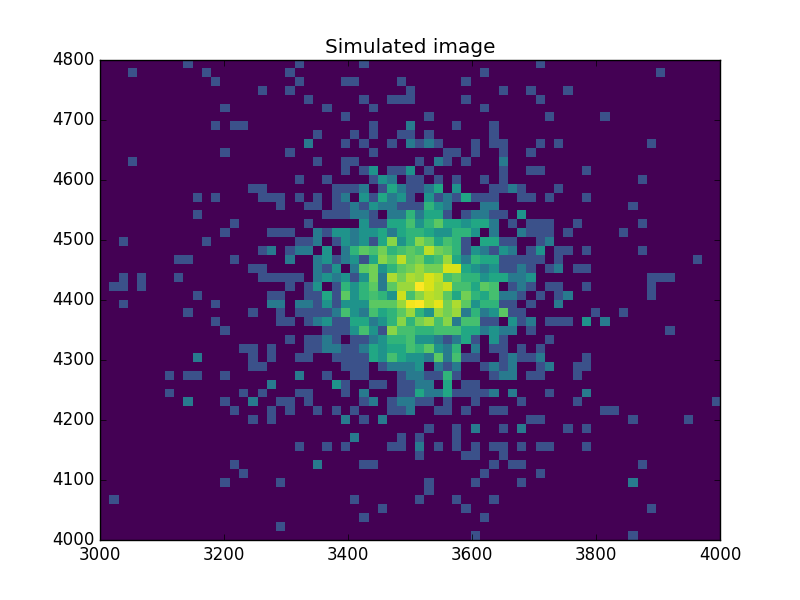### Find the starting point for the MCMC¶

Set up a model and use the standard Sherpa approach to find a good starting place for the MCMC analysis:

>>> from sherpa import data, stats, optmethods, fit
>>> d = data.Data2D('sim', x0, x1, msim.flatten(), shape=shape)
>>> mdl = Beta2D()
>>> mdl.xpos, mdl.ypos = 3500, 4400


Use a Likelihood statistic and Nelder-Mead algorithm:

>>> f = fit.Fit(d, mdl, stats.Cash(), optmethods.NelderMead())
>>> res = f.fit()
>>> print(res.format())
Statistic             = cash
Initial fit statistic = 20048.5
Final fit statistic   = 607.229 at function evaluation 777
Data points           = 3618
Degrees of freedom    = 3613
Change in statistic   = 19441.3
beta2d.r0      121.945
beta2d.xpos    3511.99
beta2d.ypos    4419.72
beta2d.ampl    12.0598
beta2d.alpha   2.13319


Now calculate the covariance matrix (the default error estimate):

>>> f.estmethod
<Covariance error-estimation method instance 'covariance'>
>>> eres = f.est_errors()
>>> print(eres.format())
Confidence Method     = covariance
Iterative Fit Method  = None
Statistic             = cash
covariance 1-sigma (68.2689%) bounds:
Param            Best-Fit  Lower Bound  Upper Bound
-----            --------  -----------  -----------
beta2d.r0         121.945     -7.12579      7.12579
beta2d.xpos       3511.99     -2.09145      2.09145
beta2d.ypos       4419.72     -2.10775      2.10775
beta2d.ampl       12.0598    -0.610294     0.610294
beta2d.alpha      2.13319    -0.101558     0.101558


The covariance matrix is stored in the extra_output attribute:

>>> cmatrix = eres.extra_output
>>> pnames = [p.split('.') for p in eres.parnames]
>>> plt.imshow(cmatrix, interpolation='nearest', cmap='viridis')
>>> plt.xticks(np.arange(5), pnames)
>>> plt.yticks(np.arange(5), pnames)
>>> plt.colorbar()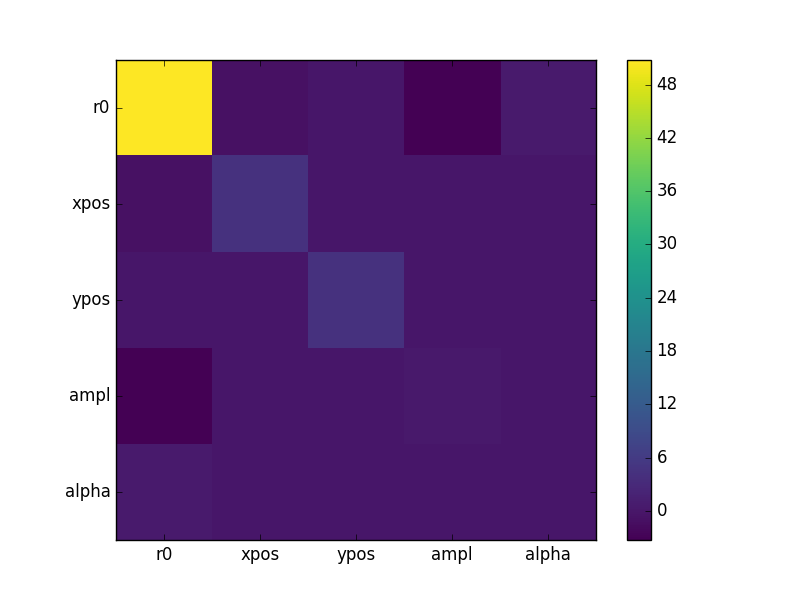### Run the chain¶

Finally, run a chain (use a small number to keep the run time low for this example):

>>> from sherpa.sim import MCMC
>>> mcmc = MCMC()
>>> mcmc.get_sampler_name()
>>> draws = mcmc.get_draws(f, cmatrix, niter=1000)
>>> svals, accept, pvals = draws

>>> pvals.shape
(5, 1001)
>>> accept.sum() * 1.0 / 1000
0.48499999999999999


### Trace plots¶

>>> plt.plot(pvals[0, :])
>>> plt.xlabel('Iteration')
>>> plt.ylabel('r0')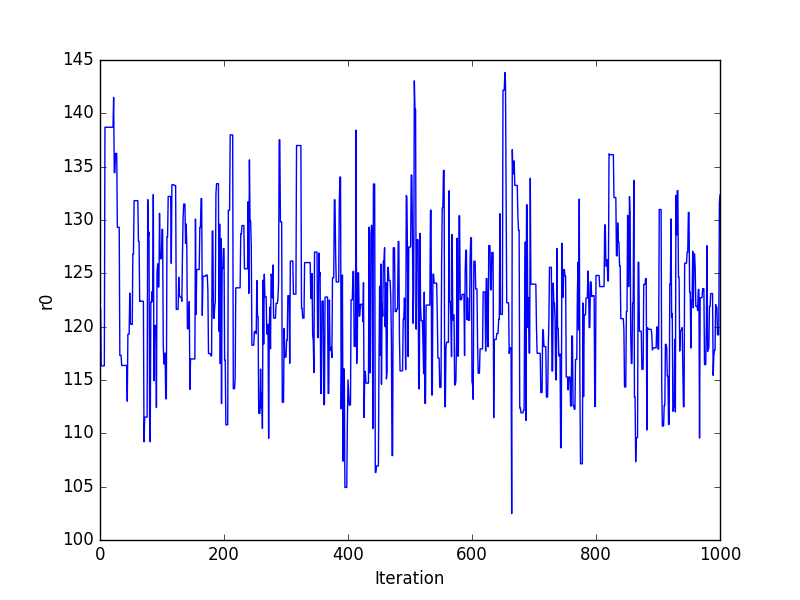Or using the sherpa.plot module:

>>> from sherpa import plot
>>> tplot = plot.TracePlot()
>>> tplot.prepare(svals, name='Statistic')
>>> tplot.plot()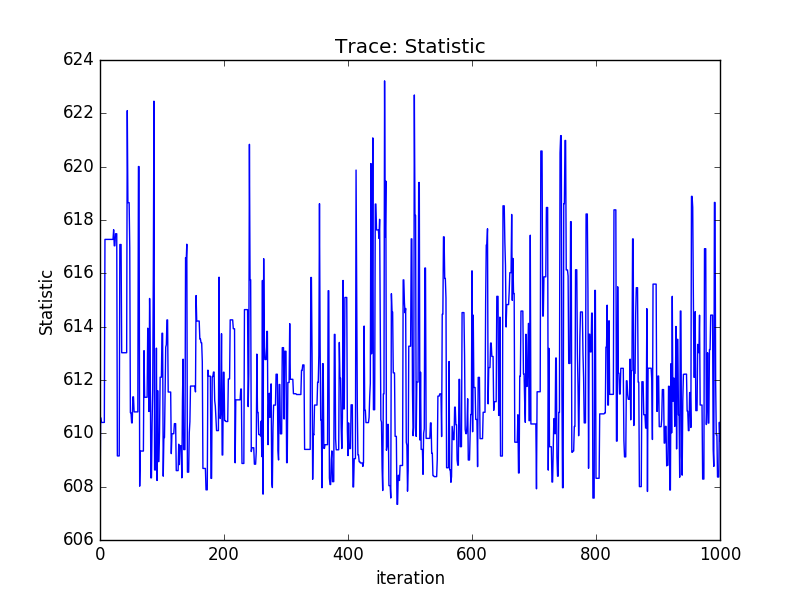### PDF of a parameter¶

>>> pdf = plot.PDFPlot()
>>> pdf.prepare(pvals[1, :], 20, False, 'xpos', name='example')
>>> pdf.plot()


>>> xlo, xhi = eres.parmins + eres.parvals, eres.parmaxes + eres.parvals

>>> plt.annotate('', (xlo, 90), (xhi, 90), arrowprops={'arrowstyle': '<->'})
>>> plt.plot([eres.parvals], , 'ok')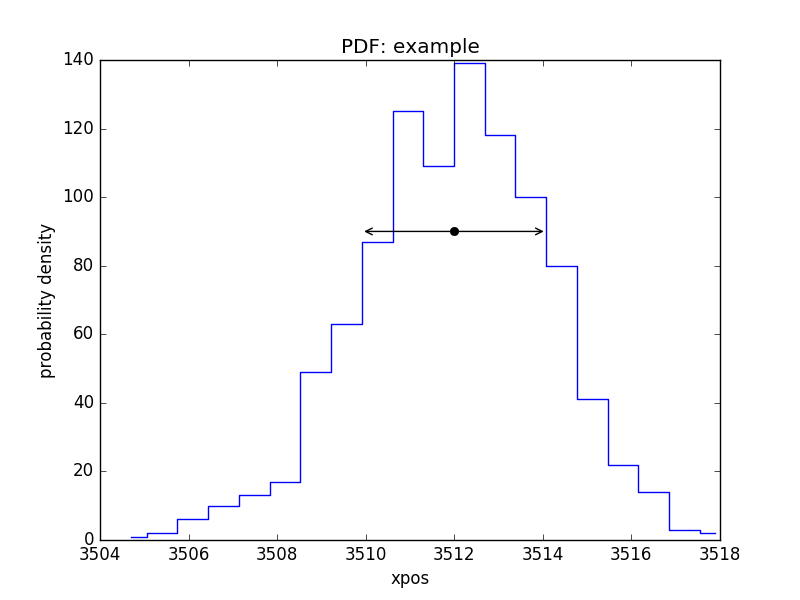### CDF for a parameter¶

Normalise by the actual answer to make it easier to see how well the results match reality:

>>> cdf = plot.CDFPlot()
>>> plt.subplot(2, 1, 1)
>>> cdf.prepare(pvals[1, :] - truth.xpos.val, r'$\Delta x$')
>>> cdf.plot(clearwindow=False)
>>> plt.title('')
>>> plt.subplot(2, 1, 2)
>>> cdf.prepare(pvals[2, :] - truth.ypos.val, r'$\Delta y$')
>>> cdf.plot(clearwindow=False)
>>> plt.title('')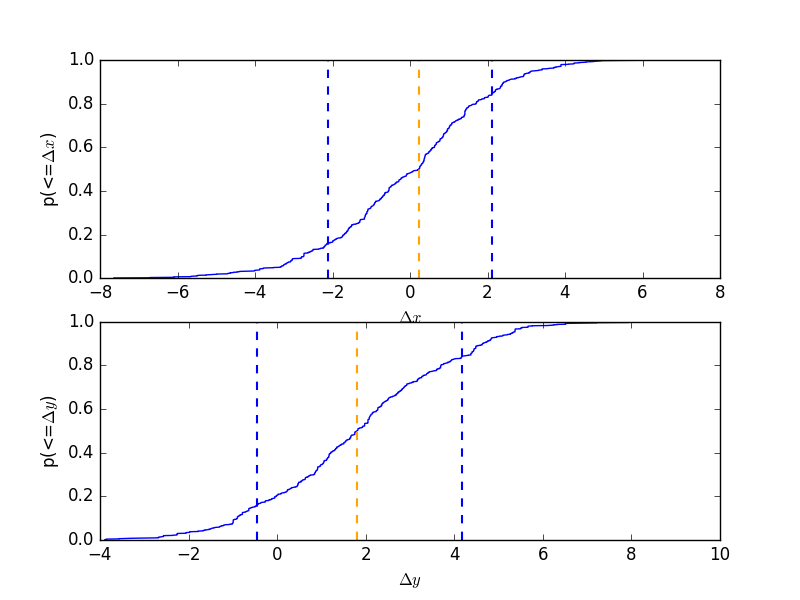### Scatter plot¶

>>> plt.scatter(pvals[0, :] - truth.r0.val,
...:             pvals[4, :] - truth.alpha.val, alpha=0.3)
>>> plt.xlabel(r'$\Delta r_0$', size=18)
>>> plt.ylabel(r'$\Delta \alpha$', size=18)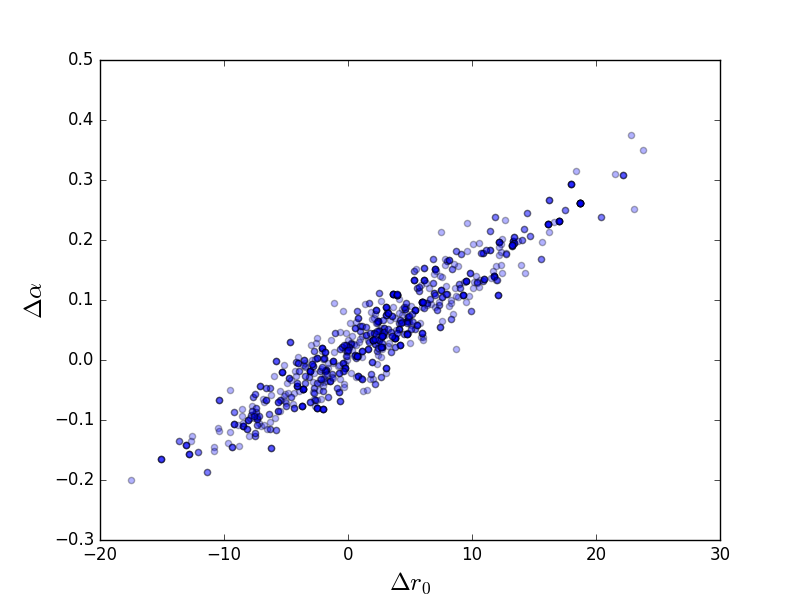This can be compared to the RegionProjection calculation:

>>> plt.scatter(pvals[0, :], pvals[4, :], alpha=0.3)

>>> from sherpa.plot import RegionProjection
>>> rproj = RegionProjection()
>>> rproj.prepare(min=[95, 1.8], max=[150, 2.6], nloop=[21, 21])
>>> rproj.calc(f, mdl.r0, mdl.alpha)
>>> rproj.contour(overplot=True)
>>> plt.xlabel(r'$r_0$'); plt.ylabel(r'$\alpha$')